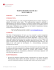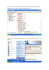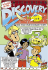# CMSC 28100-1 Spring 2015 Homework 5

## Transcription

CMSC 28100-1 Spring 2015 Homework 5
```CMSC 28100-1 Spring 2015
Homework 5
May 3, 2015
Definition
A Turing machine transducer is a Turing machine that outputs strings, rather than accepting or
rejecting. That is, if M is a Turing machine, then M computes the function which takes an input
x to the value that is left on the tape when M halts. If M does not halt on input x, then the
function computed by M is undefined on x. A function f that is computed by a Turing machine
transducer is called partial computable (“partial,” since M may not halt on some inputs, and then
f is undefined on those inputs).
Homework Questions
1.
In class/the book, you were given the following definition of “recursively enumerable:”
A language L is recursively enumerable if there is a TM M such that M accepts exactly
those strings in L. (On strings not in L, M may either reject or not halt.)
Show that each of the following conditions is equivalent to being L recursively enumerable (in
the above sense):
(a) There is a partial computable function f such that L is the range of f , that is, L = {f (x) :
x ∈ Σ∗ }. Such an f is called an enumerator of L, since when f is run on all strings in
succession, it enumerates/lists the elements of L.
(b) There is a partial computable function f such that L is the domain of f , that is, L = {x :
f (x) is defined }. Equivalently, there is a TM M such that L = {x : M halts on input x}.
2.
(a) Show the recursive analog of 1(a): L is recursive if and only if there is an enumerator f for
L such that f is monotone, that is, x < y implies f (x) < f (y) (thinking of x, y as natural
numbers). In the definition of monotonicity for partial functions, treat undefined
values of f (x) as f (x) = +∞.
(b) Show that every infinite recursively enumerable language L contains an infinite subset L0 ⊆ L
such that L0 is recursive. Hint: this is not unrelated to 1(a) and 2(a).
1
3.
Consider the following two languages:
Inf = {M |L(M ) is infinite},
and
T ot = {M |M is a total TM, that is M halts on all inputs}.
(a) Give a reduction from Inf to T ot.
(b) Give a reduction from T ot to Inf .
(c) Using the generalized/second Rice’s Theorem (a.k.a. Rice-Shapiro Theorem), show that
neither Inf nor its complement Inf are recursively enumerable.
(d) Using 3(c) and the reductions from the earlier part of this problem, show that neither T ot is
recursively enumerable.
(e) Using the Rice-Shapiro Theorem, show that neither T ot nor its complement T ot are recursively enumerable. Do this using the Rice-Shapiro Theorem directly (rather than as in 3(d)).
2
```

### CMSC 28100-1 Spring 2015 Homework 2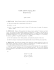### CMSC 28100-1 Spring 2015 Homework 3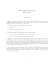### puzzle - Dallas Voice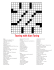### projectinfo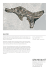### Erythrasma (Corynebacterium minutissinum)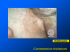### MP3. .WAV .JPG .TIFF .MOV .MPG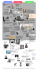### 276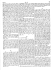### TURING PHARMACEUTICALS NEW YORK, NY